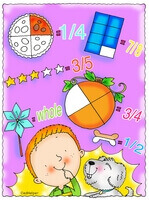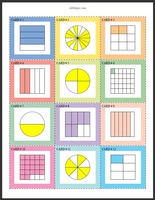Worksheets and No Prep Teaching Resources
Math Worksheets
Fraction Worksheets and Printables

# Fraction Math Learning CentersWorksheets, Lessons, and Printables

Fractions Math Center - Write the FractionWrite the fraction - numerator is always 1 (Grades 2-4) Write the fraction (Grades 3-6) Write the fraction - a blank on student page (student will need to know to draw line and where to put numerator and denominator) (Grades 3-6)

Matching - Picture of Fractions and Fractions - Math Learning Center

Matching - Picture of Fractions, Fractions, and Decimals - Math Learning Center

Ordering Numbers Math Center - Fractions

Fractions

Have a suggestion or would like to leave feedback?